Research on the Hydrodynamic and Cavitation Performance of Semi-Balanced Twisted Rudders

Jinming Ye, Di Zhang, Xianfeng Zhang, Xiaoyu Zou (2023). Research on the Hydrodynamic and Cavitation Performance of Semi-Balanced Twisted Rudders. Journal of Marine Science and Application, 22(3): 488-498. https://doi.org/10.1007/s11804-023-00350-w
 Citation: Jinming Ye, Di Zhang, Xianfeng Zhang, Xiaoyu Zou (2023). Research on the Hydrodynamic and Cavitation Performance of Semi-Balanced Twisted Rudders. Journal of Marine Science and Application, 22(3): 488-498. https://doi.org/10.1007/s11804-023-00350-w## Research on the Hydrodynamic and Cavitation Performance of Semi-Balanced Twisted Rudders

##### https://doi.org/10.1007/s11804-023-00350-w
Funds:

the National Natural Science Foundation of China under Grant 51579243

###### Corresponding author: Di Zhang E-mail: 3567438450@qq.com
• Abstract

In this study, we designed a new, semi-balanced, twisted rudder to reduce the surface cavitation problem of medium-high-speed surface warships. Based on the detached eddy simulation (DES) with the Spalart-Allmaras (SA) model (SA-DES) and the volume of fluid (VOF) method, the hydrodynamic and cavitation performances of an ordinary semi-balanced rudder and semi-balanced twisted rudder at different rudder angles were numerically calculated and compared using the commercial computational fluid dynamics (CFD) software STAR-CCM+ with the whole-domain structured grid. The calculation results showed that, under the same working conditions, the maneuverability of the semi-balanced twisted rudder basically remained unchanged compared with that of the ordinary semi-balanced rudder. Furthermore, the surface cavitation range of the semi-balanced twisted rudder was much smaller, and the inception rudder angle of the rudder surface cavitation increased by at least 5° at the maximum speed. In conclusion, the semi-balanced twisted rudder effectively reduced the cavitation of the rudder surface without reducing the rudder effect and exhibited excellent anti-cavitation performance.

Article Highlights
● A completely new semi-balanced twist rudder is designed to repress the cavitation of the rudder surface.
● The hydrodynamic and cavitation performances of the ordinary semi-balanced rudder and the semi-balanced twisted rudder are numerically calculated and compared.
● The hydrodynamic performance and anti-cavitation effect of the semi-balanced twisted rudder were evaluated.
• In large-scale surface warships and merchant ships, semi-balanced rudders are mostly used to reduce the load on the rudder shaft. The cavitation of semi-balanced rudders mainly occurs on the surface near the leading edge of the rudder blade and horn, the gap between the rudder blade and horn, and the lower end face of the rudder (Liu and Hekkenberg, 2017). Cavitation erodes the rudder surface, further influencing its service life (Liu and Hekkenberg, 2017; Li and Tom, 2013) and significantly increasing the vibration and noise at the stern of the hull. In turn, these influence the working environment of personnel, equipment, and instruments in the aft cabin of the ship and enhance the acoustic target strength of surface warships. In addition, the noise induced by rudder cavitation has prominent low and medium-frequency line spectral components with a long propagation distance. Consequently, surface warships can easily become targets of sonars and torpedoes (Grunditz et al., 2009). The anti-cavitation design of the rudder can significantly inhibit cavitation erosion of the rudder surface and reduce cavitation-induced vibration and noise at the stern of the hull.

Scholars have proposed numerous solutions by investigating rudder cavitation. For example, the rudder surface cavitation can be relieved by optimizing the rudder section, so Shen et al. (2017a, b)designed a twisted rudder to reduce the effect of rudder cavitation on destroyers. Since the 1990s, the twisted rudder scheme has gradually been applied to DDG-51 destroyers. Related to this, Choi et al. (2010) conducted numerical calculations on the twisted rudder and demonstrated its superiority to the full spade rudder in terms of speed and cavitation. Ahn et al. (2012) designed an X twisted rudder with the leading edge continuously twisted along the span and then performed numerical calculations and experiments on hydrodynamic characteristics. Their results showed that the X twisted rudder is beneficial to cavitation and maneuverability. Ye et al. (2015; 2016; 2017a, 2019; 2021) and Wang et al. (2017) designed an anti-cavitation twisted rudder that helped reduce the peak value of the negative pressure on the suction surface. Then, they established a panel method to calculate the sheet cavitation of the rudder behind the propeller and ultimately improve the calculation efficiency of rudder cavitation (Ye et al., 2019). Yu et al. (2019) conducted a cavitation observation experiment of the twisted rudder model and reported the twisted rudder's good cavitation performance. They also found that the design can improve the initial speed of rudder cavitation.

The cavitation of the semi-balanced rudder is divided in‐ to the gap and surface cavitation. In the former case, seri‐ ous fluid separation occurs above the gap, mainly due to cavitation caused by the discontinuity of the semi-bal‐ anced rudder structure, which can be repressed by reduc‐ ing the gap between the rudder horn and blade. Shin and Hyochul (2008) studied a new rudder system to improve the rudder effect and inhibit gap cavitation. Their proposed system was equipped with cam devices that effectively closed the gap between the rudder horn and movable wing parts. Oh et al. (2009) and Seo et al. (2009) found that re‐ ducing the gap flow using fluid-supplying devices or block‐ ing bars could mitigate gap cavitation. The large-area sur‐ face cavitation on the rudder blade plays an important role in the rudder cavitation. In addition, researchers have only investigated the balanced twisted rudder so far, and only a few studies have examined the cavitation characteristics and unsteady forces of the semi-balanced rudder after twist‐ ing. Therefore, the design concept of a twisted rudder was adopted in the current study to improve the semi-balanced rudder and ameliorate its anti-cavitation performance and unsteady forces. The findings of this study have great theo‐ retical significance and engineering application value for providing an effective path to inhibiting the cavitation of the semi-balanced rudder on a large-scale surface warship.

The homogeneously mixed flow model was adopted in the numerical simulation of cavitation. The modified continuity equation and momentum equation are defined as follows:

 $$\frac{\partial \rho}{\partial t}+\frac{\partial \rho u_i}{\partial x_i}=0$$ (1)
 $$\rho \frac{\partial u_i}{\partial t}+\frac{\partial \rho u_i u_j}{\partial x_j}=-\frac{\partial p}{\partial x_i}+\frac{\partial}{\partial x_j}\left(\mu \frac{\partial u_i}{\partial x_j}+\mu \frac{\partial u_j}{\partial x_i}\right)$$ (2)
 $$\rho=\alpha \rho_v+\rho_l(1-\alpha)$$ (3)
 $$\rho=\alpha \rho_v+\rho_l(1-\alpha)$$ (4)

where xi and xj represent the Cartesian coordinate components; ui and uj are the velocity vectors; ρ, μ, and ρ are the density, dynamic viscosity, and pressure of the mixed phase, respectively; μ, is the turbulence viscosity; and α represents the vapor volume fraction. Finally, the subscripts l and v represent the liquid and vapor phases, respectively.

The Schnerr–Sauer model (Schnerr and Sauer, 2001) based on the Rayleigh–Plesset equation was adopted in the cavitation calculation; in this model, all bubbles were considered spherical with the same radius at birth. The following evaporation and condensation terms are used to describe the mass conversion rate between phases:

 $$m_e=\frac{\rho_v \rho_l}{\rho} \frac{3 \alpha(1-\alpha)}{R} \sqrt{\frac{2}{3} \frac{p_v-p}{\rho_l}}, p_v \geqslant p$$ (5)
 $$m_c=-\frac{\rho_v \rho_l}{\rho} \frac{3 \alpha(1-\alpha)}{R} \sqrt{\frac{2}{3} \frac{p_v-p}{\rho_l}}, p_v The vapor bubble radius R is given by: $$ R=\left(\frac{\alpha}{1-\alpha} \frac{3}{4 \pi} \frac{1}{n_0}\right)^{1 / 3} $$(7) To select an appropriate turbulence model based on structured grids and the VOF method, the sheet cavitation of a three-dimensional (3D) twisted hydrofoil was calculated using SST-k-ω and the SA-DES turbulence model (Gonstantinescu and Squires, 2003; Spalart et al., 1997; Spalart 2000). This 3D hydrofoil had a chord length of 0.15 m and 0.3 m in extension, with the section being a NACA0009 airfoil profile (Cao et al., 2014; Cao et al., 2017; Ji et al., 2013). The grid number of the 3D hydrofoil was 1.57 million, and all y+ wall treatment functions were employed. The cavitation number was set as 1.07, and the outlet pressure was set as 29 484 Pa to ensure consistency with the experimental conditions. The comparison between the calculation and the experimental results of the sheet cavitation of 3D hydrofoil is presented in Figure 1, where αv > 0.1 indicates the occurrence of cavitation. As shown in the figure, only the rough range of sheet cavitation was calculated by the SST-k-ω turbulence model, thus failing to forecast cavitation shedding. Meanwhile, the sheet cavitation area and the shedding situation of cavitation calculated by the SA-DES turbulence model showed good agreement with the experimental results. Hence, the SA-DES turbulence model was selected in the present study to calculate the cavitation of the rudder behind the propeller.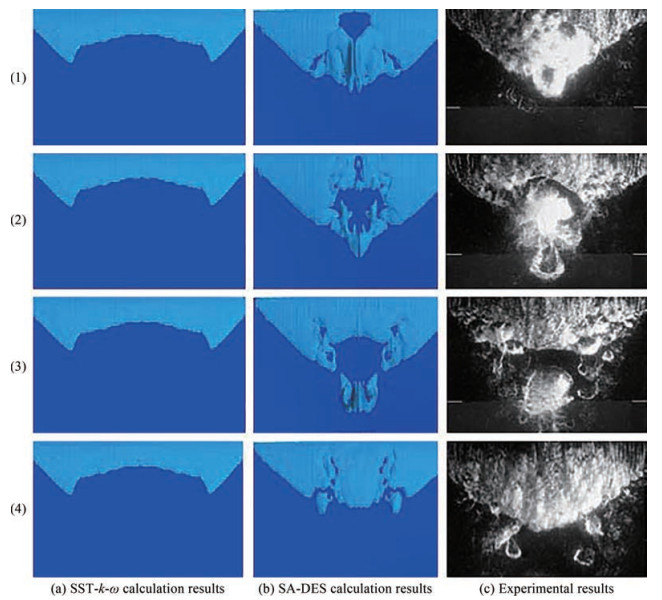Figure 1 Comparison of sheet cavitation of a 3D twisted hydrofoil To validate the calculation accuracy of the selected method, the open-water hydrodynamic performance of a propeller was evaluated. A comparison between the calculated and experimental values of the propeller is shown in Figure 2. As can be seen, the calculation error of the thrust coefficient can be maintained within 4% when the advance coefficient J < 1.2, whereas the calculation error of the torque coefficient and open-water efficiency can be controlled within 5%. Therefore, the numerical method is deemed reliable in terms of the calculation accuracy considered in the present study.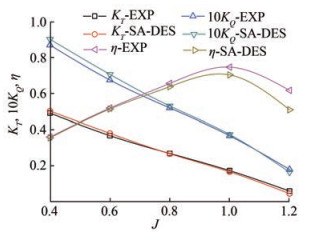Figure 2 Open-water performance of the propeller We established the propeller and two rudder models of a large-scale surface warship at the model scale. The surface warship is a twin-screw ship with inward-turning propellers, and the left rudder and propeller are taken as research objects. When designing the semi-balanced twisted rudder, we retained the chordwise thickness distribution in the section at each spanwise position, and the camber was set to zero. Next, we calculated the hydrodynamic performance of the rudder using the finite-volume method. Then, we obtained the pressure distributions on the pressure and suction surfaces on each section of the rudder and considered the spanwise distribution of the geometric angles of attack as the adjustment parameter. The objective function was the quadratic sum of the pressure difference between the pressure and suction surfaces at all specified chordwise positions in each section. The corresponding data that enabled the objective function of each section to reach the minimum value was solved using the least squares method. Then, the rudder was twisted according to the obtained spanwise distribution data of the geometric angle of attack. After the completion of the design, we separated the gaps in each section to form a semi-balanced twisted rudder, after which we compared the geometry models of the two types of rudders. The results are shown in Figure 3.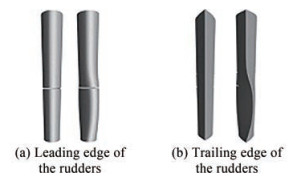Figure 3 Comparison of two rudder models The computational domain consisted of three parts: rotation, rudder, and outer domains (see Figure 4). Information transmission between domains was achieved by setting the interfaces. In the Figure 4, D represents the diameter of the propeller. Cavitation flow is an unsteady flow that imposes higher requirements for grid division. Related to this, structured grids have been extensively used in cavitation simulations due to their advantages, such as high quality and the ability to efficiently simulate boundary layers by setting the node number. Therefore, this study adopted whole-domain structured grids for simulation (Figure 5), and the number of grids in the entire computational domain was set as 24.2 million.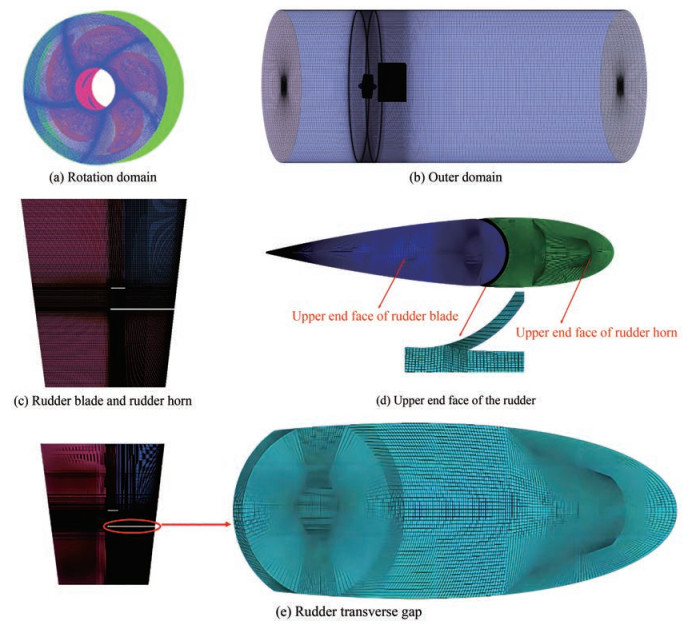Figure 5 Grids of the computational domain For the calculated rudder angles of 0°, 3°, 5°, 7°, and 10°, the inflow velocity and rotational speed of the propeller under the model scale were determined as 5.72 m/s and 1 200 r/min, respectively, based on the advance coefficient of the full-scale ship. This condition corresponds to a full-scale ship sailing at maximum speed. The outlet pressure was set to 0 Pa during the hydrodynamic numerical simulation. The pressure coefficient distributions on the inner surface of the two rudders at different rudder angles are shown in Figure 6. Here, we defined the inner and outer surfaces of the rudder blade as the suction and pressure surfaces, respectively. The cloud figures of the pressure distribution showed evidently higher pressure on the suction surface of the semi-balanced twisted rudder than that of the ordinary semi-balanced rudder at each angle.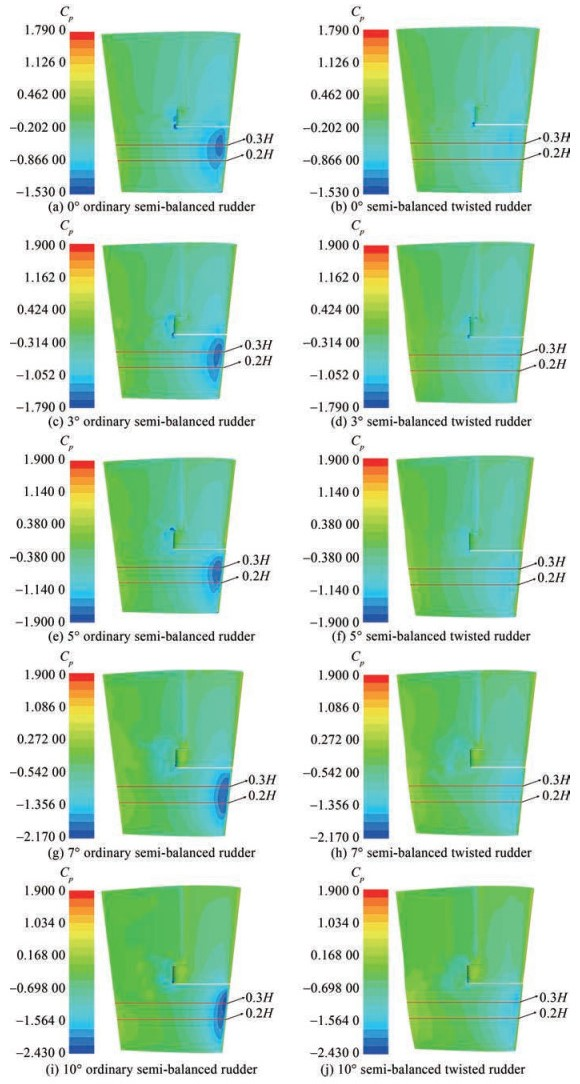Figure 6 Comparison of the pressure distributions of two rudders at different rudder angles The pressure was determined using the dimensionless method expressed by the following equation: $$ C_p=\frac{p-p_0}{0.5 \rho n^2 D^2} $$(8) where ρ, n, D, and ρ represent water density, propeller speed, propeller diameter, and absolute pressure, respectively. Here, p0 represents the reference pressure (hydrostatic pressure at the distant front of the rudder) and can be obtained using the following equation: $$ p_0=p_a+\rho g h  (9)

where pa represents the standard atmospheric pressure. As no gravity model is introduced during the simulation, ρgh equals zero; that is, p0 = pa.

The pressure distributions at different rudder heights were compared to clearly distinguish the pressure distributions of the semi-balanced twisted rudder and semi-balanced rudder. Specifically, the pressure coefficient distributions on the section at the two rudder height positions of y=0.2H and y=0.3H were compared, where H represented the rudder height.

The pressure distribution curves of the two sections of the ordinary semi-balanced rudder and semi-balanced twisted rudder at rudder angles of 0°, 3°, 5°, 7°, and 10° at an inflow velocity of 5.72 m/s and a rotational speed of 1 200 r/min are presented in Figure 7. As shown in the figure, C represents the chord length at different spanwise sections, x represents the chordwise distance from the leading edge to the point on the section, and x/C denotes the chordwise position of the point on the section relative to the chord length. Here, the ordinary rudder is an ordinary semi-balanced rudder, and the twisted rudder is a semi-balanced twisted rudder.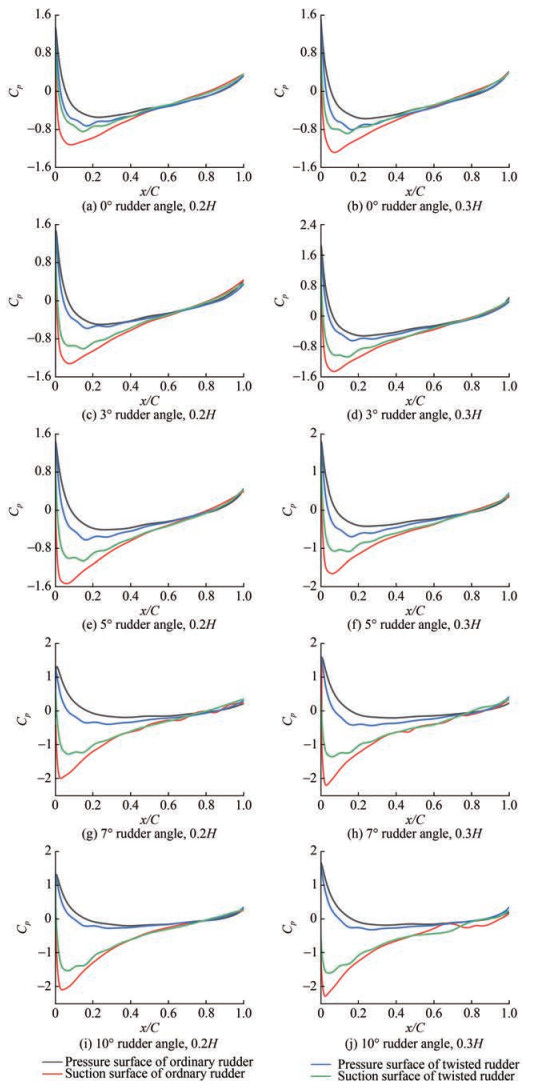Figure  7  Pressure coefficient distributions on two sections of rudders

Table 1 lists the peak values of the pressure coefficient of the two sections at different rudder angles. Meanwhile, Figure 7 and Table 1 present the reduction in the peak value of the negative pressure coefficient of the semi-balanced twisted rudder compared with the ordinary semi-balanced rudder at the same rudder angle. For example, the peak values of the negative pressure coefficient of the semi-balanced rudder and semi-balanced twisted rudder for a rudder angle of 5° were 1.665 and 1.095, respectively, in which the latter was 34.2% lower than the former. This result indicates that the semi-balanced twisted rudder can effectively ameliorate the cavitation performance of the semi-balanced rudder and increase the inception rudder angle of surface cavitation.

Table  1  Comparison between the negative pressure peak values of the two kinds of rudders at different rudder angles
 Rudder angle (°) Rudder type Peak values of the pressure coefficient 0.2H 0.3H 0 Ordinary semi-balanced rudder -1.127 -1.290 Semi-balanced twisted rudder -0.848 -0.890 3 Ordinary semi-balanced rudder -1.318 -1.454 Semi-balanced twisted rudder -1.004 -1.074 5 Ordinary semi-balanced rudder -1.536 -1.665 Semi-balanced twisted rudder -1.065 -1.095 7 Ordinary semi-balanced rudder -2.003 -2.211 Semi-balanced twisted rudder -1.283 -1.362 10 Ordinary semi-balanced rudder -2.103 -2.293 Semi-balanced twisted rudder -1.539 -1.610

By comparing the pressure distribution curves of different sections of the ordinary semi-balanced and semi-balanced twisted rudders, it can be observed that the peak value of the negative pressure on the twisted rudder was smaller. Therefore, the semi-balanced twisted rudder suppressed the cavitation on the rudder surface. Furthermore, the peak values of the negative pressure coefficient at all rudder angles were always maximum at a section of 0.3H. To explore the main reason for such a phenomenon, the interaction between the hull and rudder was neglected, and the computational domain for the open-water area of the propeller model was established. We arranged several monitoring points at the installation position of the rudder shaft (Figure 8) to monitor the transverse and axial velocities at these points. After the simulation stabilized, we then determined the time-averaged values of the unsteady transverse and axial velocities at these points within one propeller period, as shown in Figure 9. In addition, we determined the inflow angles of attack at different positions (Figure 10). As shown in Figure 9 and 10, the respective axial and transverse velocities of the propeller wake flow at 0.3H nearly reached the maximum values, and the inflow angle of attack was also the largest.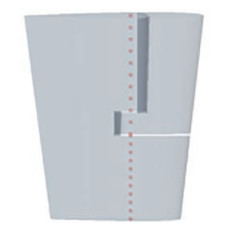Figure  8  Layout of the speed monitoring points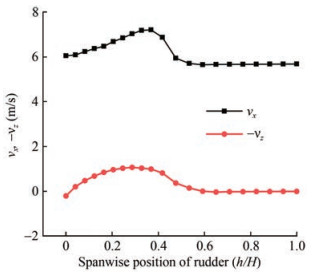Figure  9  Time-average values of inflow velocity at different monitoring points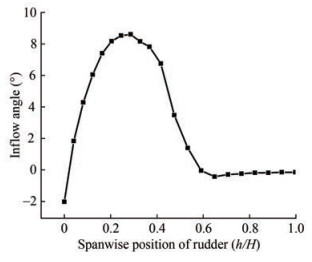Figure  10  Time-averaged angles of inflow attack at different monitoring points

The pressure on the suction surface of the semi-balanced twisted rudder almost overlaps with that on the pressure surface and presents a symmetrical pattern at the rudder angle of 0°. In turn, this results in a smaller peak value of the negative pressure coefficient and significantly reduces the transverse force compared with that on the ordinary semi-balanced rudder when the ship is in straight-ahead cruising mode. To further analyze the influence of the twisted rudder on maneuverability, we monitored the transverse force and torque of the two rudders during the CFD simulation. Considering the left rudder as the study object, the interaction between the left and right rudders was neglected. Once the calculation stabilized, we were able to obtain the time-averaged values of the unsteady transverse force and torque of the two rudders within a single propeller period were obtained. The force and torque of the two rudders at different rudder angles are listed in Table 2, where Fy represents the rudder force along the transverse direction of the ship pointing from the amidships to the starboard (unit: N), and Mz represents the torque of the rudder shaft, which is positive in the clockwise direction (N⋅m).

Table  2  Rudder force and torque of two rudders with different rudder angles
 Rudder angle (°) Ordinary semi-balanced rudder Semi-balanced twisted rudder Fy(N) Mz(N·m) Fy(N) Mz(N·m) -10 -163.27 1.584 -208.73 2.556 -7 -99.38 1.195 -144.68 1.897 -5 -58.09 0.682 -104.25 1.514 -3 -10.85 0.124 -59.73 0.824 0 55.14 -1.232 1.63 -0.162 3 112.47 -1.654 62.66 -0.841 5 150.71 -2.031 105.71 -1.545 7 187.66 -2.629 145.36 -1.946 10 247.75 -3.313 209.69 -2.695

As seen in Table 2, due to the existence of a transverse component in the propeller wake flow, the ordinary semi-balanced rudder still exhibited a large transverse force (55.14 N) and torque of the rudder shaft (–1.23 N⋅m) at a rudder angle of 0°. However, the shaft torque and transverse force of the semi-balanced twisted rudder were –0.162 N⋅m and 1.63 N, respectively. A subsequent comparison revealed that the transverse force and shaft torque were nearly reduced by one order of magnitude at the rudder angle of 0° after the semi-balanced twisted rudder was used. The semi-balanced twisted rudder can obviously ameliorate the unsteady forces of the steering gear during the straight-ahead cruising mode. In addition, the maximum shaft torque of the ordinary semi-balanced rudder was 3.313 N ⋅ m, whereas that of the semi-balanced twisted rudder was only 2.695 N⋅m. Therefore, the twisted rudder well reduced the load of the steering gear.

To further compare the maneuverability of the semi-balanced and semi-balanced twisted rudders, the total transverse force of the rudder was obtained by subtracting the transverse force generated when the rudder turned left and that when it turned right. The calculation results for the two rudders with different angles are listed in Table 3.

Table  3  Total transverse force
 Rudder angle (°) Ordinary semi-balanced rudder (N) Semi-balanced twisted rudder (N) Difference (%) 3 123.32 122.39 -0.76 5 208.8 209.96 0.55 7 287.04 290.04 1.03 10 411.02 418.42 1.77

As shown in Table 3, within a rudder angle of 10°, the difference between the total transverse forces of the ordinary semi-balanced rudder and the semi-balanced twisted rudder was within 2%. Thus, the two rudders exhibited equivalent maneuverability.

The presence of cavitation on the rudder surface can be determined according to the correlation between the minimum pressure coefficient of the rudder surface (Cpmin) and the cavitation number (σv). In particular, there is an absence of cavitation when - Cpmin < σv, namely, pmin > pv, and cavitation is present when - Cpminσv, namely, pminpv, where pmin denotes the minimum pressure on the rudder surface, and pv is the saturated vapor pressure.

Here, we calculated the cavitation number of a full-scale ship using σS = 1.143. By combining Table 1, cavitation was generated on the rudder surface even at a rudder angle of 0° for the ordinary semi-balanced rudder, whereas no surface cavitation was generated for the semi-balanced twisted rudder at a rudder angle of 5°, thereby indicating an increase in the cavitation inception rudder angle by at least 5° by designing the semi-balanced twisted rudder.

Hereby, at an inflow velocity of 5.72 m/s and a rotational speed of 1 200 r/min, the cavitation of the semi-balanced twisted rudder with different rudder angles was predicted through CFD simulations to obtain more detailed data. Then, we compared these with those of the ordinary semi-balanced rudder to verify the cavitation performance of the semi-balanced twisted rudder, as shown in Figure 11 (the left side is the semi-balanced rudder, and the right side is the semi-balanced twisted rudder). Given that the cavitation range and volume on the rudder surface change over time, we display those moments when the cavitation volume at the suction surfaces of the rudders was at the maximum. In the figure, cavitation is indicated by the light-blue region.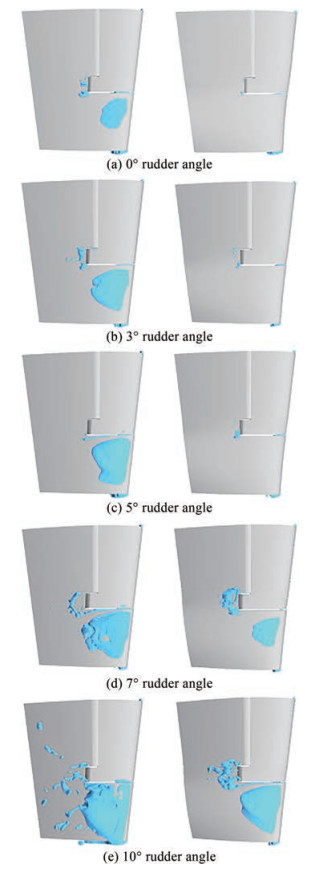Figure  11  Comparison of the cavitation of two rudders with different rudder angles

Figure 11 shows that the cavitation inception rudder angle for the semi-balanced twisted rudder is evidently larger than that of the ordinary semi-balanced rudder. At the maximum navigational speed, the ordinary semi-balanced rudder-experienced cavitation was just at the rudder angle of 0°, while it was absent at a rudder angle of 5° for the semi-balanced twisted rudder. Furthermore, at the rudder angle of 7°, cavitation materialized on the surface of the semi-balanced twisted rudder, but the cavitation range was much smaller than the semi-balanced rudder at the same angle.

The above calculation results indicate that the semi-balanced twisted rudder can increase the range of the non-cavitation rudder angle at the maximum navigational speed. In addition, even if cavitation occurs, the cavitation range of the semi-balanced twisted rudder is evidently smaller than that of an ordinary semi-balanced rudder. Therefore, the semi-balanced twisted rudder enhances its anti-cavitation performance.

With the aim of addressing the cavitation problem of a semi-balanced rudder, this study improved the original rudder by designing a new semi-balanced twisted rudder. Then, its hydrodynamic and cavitation performances were analyzed through CFD. The following conclusions were drawn:

1) The ordinary semi-balanced rudder has different inflow angles of attack at different rudder heights. This can easily produce a low-pressure zone in an area with large velocities and inflow angles of attack, which leads to cavitation.

2) We designed a new type of semi-balanced twisted rudder based on the inflow angles of attack at different rudder heights. On this basis, the rudder better matched the surrounding flow field, and the peak values of the negative pressure of the semi-balanced twisted rudder at different rudder angles were evidently reduced compared with the ordinary rudder. The inception rudder angle of the rudder's surface cavitation increased by at least 5° at the maximum speed, effectively improving the anti-cavitation performance of the rudder.

3) At a rudder angle of 0°, the pressure on the suction surface was lower than that of the ordinary semi-balanced rudder. However, the pressure distribution on the suction surface almost overlapped with that on the pressure surface of the semi-balanced twisted rudder. Owing to this feature, the peak value of the twisted rudder's negative pressure obviously reduced, the transverse force of the semi-balanced twisted rudder became much smaller than that of the ordinary semi-balanced rudder during straight-ahead cruising, and the load of the steering gear was effectively reduced.

4) The semi-balanced twisted rudder can considerably improve the anti-cavitation performance without degrading the rudder effect. Therefore, it is of great theoretical significance and engineering application value.

5) It can be found through simulations that cavitation also occurs in the gap of the semi-balanced rudder; for further research, the influence of the semi-balanced twisted rudder on gap cavitation can be studied in detail. On this basis, the semi-balanced twisted rudder can be further optimized to achieve a better overall anti-cavitation performance.

Competing interest  The authors have no competing interests to declare that are relevant to the content of this article.
• Figure  1   Comparison of sheet cavitation of a 3D twisted hydrofoil

Figure  2   Open-water performance of the propeller

Figure  3   Comparison of two rudder models

Figure  4   Computational domain

Figure  5   Grids of the computational domain

Figure  6   Comparison of the pressure distributions of two rudders at different rudder angles

Figure  7   Pressure coefficient distributions on two sections of rudders

Figure  8   Layout of the speed monitoring points

Figure  9   Time-average values of inflow velocity at different monitoring points

Figure  10   Time-averaged angles of inflow attack at different monitoring points

Figure  11   Comparison of the cavitation of two rudders with different rudder angles

Table  1   Comparison between the negative pressure peak values of the two kinds of rudders at different rudder angles

 Rudder angle (°) Rudder type Peak values of the pressure coefficient 0.2H 0.3H 0 Ordinary semi-balanced rudder -1.127 -1.290 Semi-balanced twisted rudder -0.848 -0.890 3 Ordinary semi-balanced rudder -1.318 -1.454 Semi-balanced twisted rudder -1.004 -1.074 5 Ordinary semi-balanced rudder -1.536 -1.665 Semi-balanced twisted rudder -1.065 -1.095 7 Ordinary semi-balanced rudder -2.003 -2.211 Semi-balanced twisted rudder -1.283 -1.362 10 Ordinary semi-balanced rudder -2.103 -2.293 Semi-balanced twisted rudder -1.539 -1.610

Table  2   Rudder force and torque of two rudders with different rudder angles

 Rudder angle (°) Ordinary semi-balanced rudder Semi-balanced twisted rudder Fy(N) Mz(N·m) Fy(N) Mz(N·m) -10 -163.27 1.584 -208.73 2.556 -7 -99.38 1.195 -144.68 1.897 -5 -58.09 0.682 -104.25 1.514 -3 -10.85 0.124 -59.73 0.824 0 55.14 -1.232 1.63 -0.162 3 112.47 -1.654 62.66 -0.841 5 150.71 -2.031 105.71 -1.545 7 187.66 -2.629 145.36 -1.946 10 247.75 -3.313 209.69 -2.695

Table  3   Total transverse force

 Rudder angle (°) Ordinary semi-balanced rudder (N) Semi-balanced twisted rudder (N) Difference (%) 3 123.32 122.39 -0.76 5 208.8 209.96 0.55 7 287.04 290.04 1.03 10 411.02 418.42 1.77click to enlarge
Figures(11)  /  Tables(3)
##### Publishing history
• Accepted:  16 November 2022

### 目录/

• Share
•Scan QR code with wechat

Share with friends and circles of friends